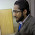## Monday, June 27, 2011

### Plotting rooted trees

Now that I have a function to generate stochastic character mapped trees, I thought I would try and see if there would be an easy way to plot them - that is without having to first transform the tree object into another format. [For instance, if I printed the tree to file, I could then read it into FigTree, which I believe can plot mutationally mapped trees; similarly, Rich Fitzjohn tells me that {diversitree} has SIMMAP plotting capabilities.]

To venture down this road, I first had to try to figure out how trees are plotted. I'll be the first to admit that this is largely untrodden territory for me (with the possible exception of my phylomorphospace plotting function). This was this morning's adventure, and I have just posted a function, plotTree(), which (if not pretty) seems to do the trick.

A few comments on the process. First, getting the lengths of edges, as well as their horizontal positions, is easy. In fact, I had already solved this in an earlier function. Second, the tricky part was figuring out vertical spacing and ordering. Some clues about how to do this are helpfully provided in Felsenstein's (2004) book chapter on plotting trees. This proved to be invaluable in the end.

The way we do this is first by reordering the tree "cladewise" (using reorder.phylo() from the {ape} package) and then spacing the tips of the tree out evenly. The height of all the terminal edges is now assigned. Next, we reorder the tree "pruningwise" - this allows us to now go from the tips of the tree to the root, encountering each internal node before its predecessor. Each time, we assign a vertical position for that node (and the corresponding preceding edge) as the average position of the two descendants, in a binary tree (or the average of the first and the last in a tree with multifurcations). This is the "intermediate" algorithm given by Felsenstein (2004; p. 574) Finally, we connect all the horizontal edges with vertical lines, and add tip labels if inclined.

Even though this plotting function just does more or less the same thing (but slower) as {ape}'s plot.phylo(), I have posted the code here for interested readers. Example execution code and plot are given below:

> require(ape)
> source("plotTree.R")
> tree<-rtree(25)
> plotTree(tree)#### 1 comment:

1.This comment has been removed by the author.

Note: due to the very large amount of spam, all comments are now automatically submitted for moderation.